##### GMAT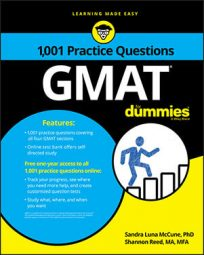Some Problem Solving questions in the Quantitative section of the GMAT will involve algebra. You should be prepared to deal with polynomials, linear equations and inequalities, quadratic equations, basic function concepts, and systems of linear equations.

## Practice questions

1. Given the function,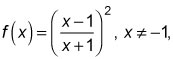which of the following expressions is equivalent to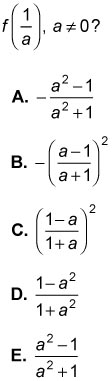2. What is the maximum value of P = 2x + 5y subject to the constraints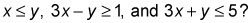A. 3.5 B. 8.75 C. 12 D. 12.25 E. 14

1. The correct answer is C.

Find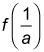and simplify.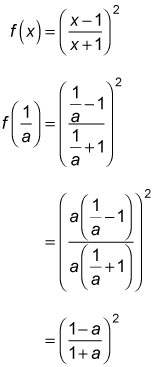2. The correct answer is C.

The three constraint inequalities define a region in the xy-plane that represents their intersections. The region's boundary equations are x = y, 3xy = 1, and 3x + y = 5. The maximum value of P will occur at one of the intersections of these three linear equations. To find the maximum value of P, systematically pair the three equations and solve for their intersections. Solving x = y and 3xy = 1 by substitution yields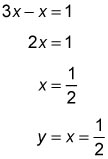Thus, the intersection of x = y and 3xy = 1 is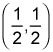Solving x = y and 3x + y = 5 by substitution yields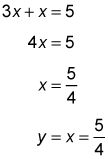Thus, the intersection of x = y and 3x + y = 5 is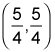Solving 3xy = 1 and 3x + y = 5 by elimination yields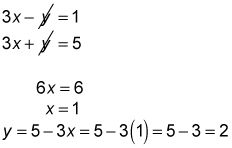Thus, the intersection of 3xy = 1 and 3x + y = 5 is (1,2). Substitute the intersection points into P = 2x + 5y to find the maximum: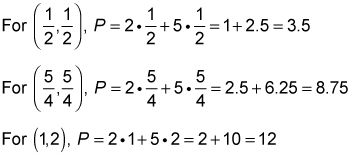Therefore, subject to the given constraints, the maximum value of P is 12, which occurs at (1,2).